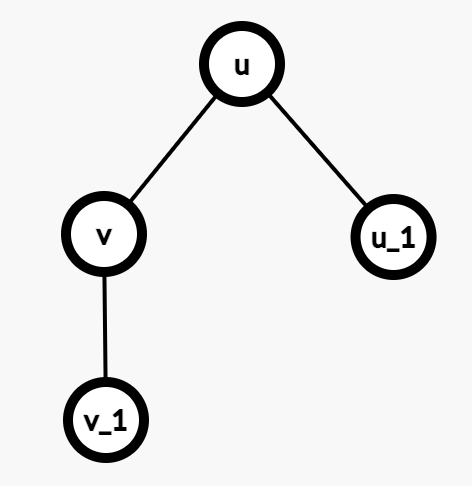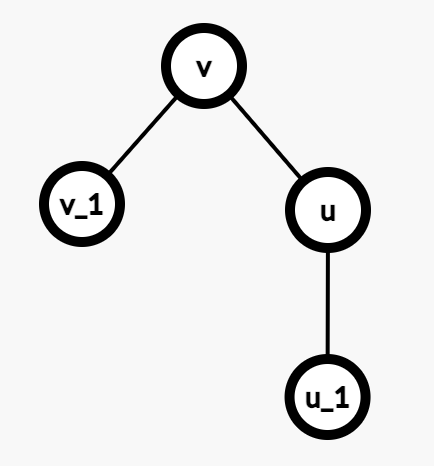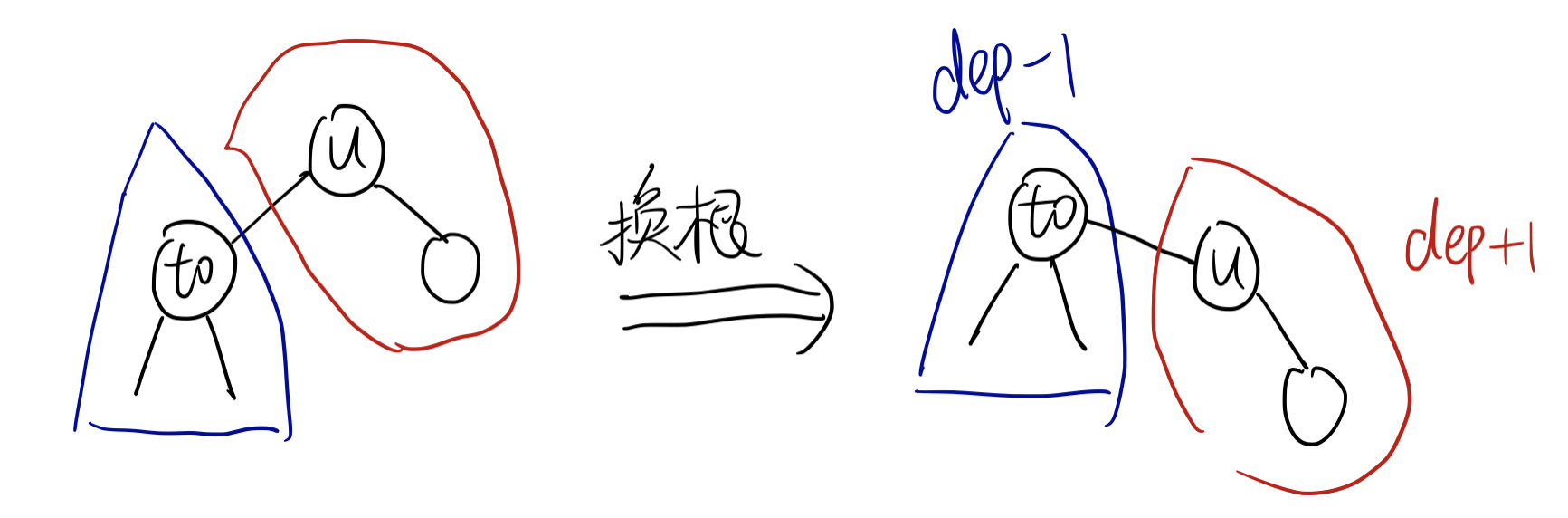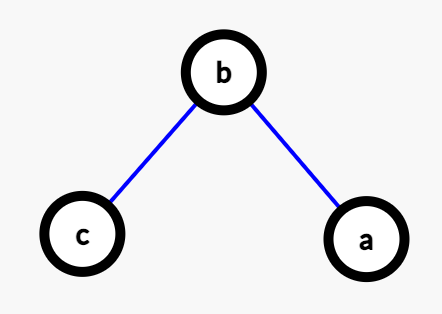## 套路1. 基于 $ans[u]$，将 $u$ 的child $v$ 所带来的贡献删掉，得到 $dp2[u]$。
2. 基于 $dp[v]$，将 $u$ 作为一个新的child 加给 $v$（实际上，就是将 $dp2[u]$ 的贡献加给 $dp[v]$），得到 $ans[v]$。

• 注意，这里的 $dp2[u]$ 实际上是一个临时的变量。对于每一个 $v$ 而言，$dp2[u]$ 互不相同。

## 例题

### 例1 洛谷P3478 [POI2008]STA-Station

dp[u] 为第一次DFS，只考虑 $u$ 的子树内的答案。（考虑深度和即可）

ans[u] 为最终答案，那么有 ans = dp#include <bits/stdc++.h>
using namespace std;
const int maxn = 1e6+5;

struct Edge {
int to, nxt;
} edges[maxn<<1];
int n, head[maxn], ecnt = 1;

void addEdge(int u, int v) {
edges[ecnt++] = e;
}

ll sz[maxn], dp[maxn];
void dfs1(int u, int p) {
sz[u] = 1;
for (int e = head[u]; e; e = edges[e].nxt) {
int to = edges[e].to;
if (to == p) continue;
dfs1(to, u);
sz[u] += sz[to];
dp[u] += dp[to] + sz[to];
}
}

ll ans[maxn];
void dfs2(int u, int p) {
for (int e = head[u]; e; e = edges[e].nxt) {
int to = edges[e].to;
if (to == p) continue;
ans[to] = ans[u] - sz[to] + ((ll)n - sz[to]);
dfs2(to, u);
}
}

int main() {
cin >> n;
for (int i = 1; i <= n-1; i++) {
int u,v; cin >> u >> v;
}
dfs1(1, 0);
ans = dp;
dfs2(1, 0);

ll maxans = 0, idx = 0;
for (int i = 1; i <= n; i++) {
if (ans[i] > maxans) {
maxans = ans[i];
idx = i;
}
}
cout << idx << endl;
}


### 例2 CF1324F Maximum White Subtree

1. to 不使用外面的节点：$ans[to] = \max(ans[to], dp[to])$

2. to 使用外面的节点：分两种情况讨论

1. 如果 to 已经被包含在 $u$ 的答案中了（used[to] = 1），则 $ans[to] = \max(ans[to], ans[u])$
2. 如果 to 并没有被包含在 $u$ 的答案中，（used[to] = 0），那么 to 的最终答案，就是由 $to$ 的subtree 和 外面节点的合并而来。即 $ans[to] = \max(ans[to], ans[u] + dp[to])$

#include <bits/stdc++.h>
using namespace std;
const int maxn = 2e5+5;

struct Edge {
int to, nxt;
} edges[maxn<<1];
int n, head[maxn], ecnt = 1, val[maxn];

void addEdge(int u, int v) {
edges[ecnt++] = e;
}

int dp[maxn];
bool used[maxn];  // when calculating answer, whether used[u] is taken into consideration
void dfs1(int u, int p) {
dp[u] = val[u];
for (int e = head[u]; e; e = edges[e].nxt) {
int to = edges[e].to;
if (to == p) continue;
dfs1(to, u);
if (dp[to] > 0) dp[u] += dp[to], used[to] = 1;
}
}

int ans[maxn];
void dfs2(int u, int p) {
ans[u] = max(ans[u], dp[u]);
for (int e = head[u]; e; e = edges[e].nxt) {
int to = edges[e].to;
if (to == p) continue;
if (used[to]) ans[to] = max(ans[to], ans[u]);
else ans[to] = max(ans[to], ans[u] + dp[to]);
dfs2(to, u);
}
}

int main() {
cin >> n;
for (int i = 1; i <= n; i++) {
cin >> val[i];
if (val[i] == 0) val[i] = -1;
}
for (int i = 1; i <= n-1; i++) {
int u,v; cin >> u >> v;
}
fill(ans, ans+maxn, -1e9);
dfs1(1,0);
dfs2(1,0);
for (int i = 1; i <= n; i++) cout << ans[i] << " ";
cout << endl;
}


### 例3 洛谷P6419 [COCI2014-2015#1] Kamp

• 以下，所有初始点我们都打上标记。

if (sz[to])
dp[u] += dp[to] + 2*w;


1. $to$ 内无标记：

先从 $to$ 走到 $u$，访问所有的点，再从 $u$ 回到 $to$。

ans[to] = ans[u] + 2LL * w;
d[to] = d[u] + w;  // 现在，d[] 表示全局的链

2. $to$ 里面包含了整棵树的所有标记点：

最终答案就 等于 以 $to$ 为根，subtree的答案。

ans[to] = dp[to];

3. $to$ 里面包含了标记，外面也包含了标记：

那么对于整棵树而言，从 $to$ 出发，还是从 $u$ 出发都一样。所以 ans[to] = ans[u]

但是我们需要更新最长链和次长链。这个时候，我们就要分类讨论 f[u] = to 与否。

如果 $u$ 原本的最长链就不在 $to$ 的子树内，那么换根以后（旋转），$to$ 的最长链必然是 $to \rightarrow u \rightarrow f[u]$。

如果 $u$ 原本用到的最长链是 $to$，而旋转后，$to$ 的最长链就有可能用到 $u$ 的其他子树（除了 $to$ 以外的子树），所以我们需要维护次长链 $s[u]$，并且进行比较。

相应的，换根过程中，我们也要更新次长链 $s[to]$。

 ans[to] = ans[u];
if (f[u] != to) {  // 原本 to 不是最长，那么现在也必不可能是最长，所以 to 起点的最长链必然继承 u 原来的最长链
s[to] = d[to];
d[to] = w + d[u];
f[to] = u;
} else {
if (s[u] + w >= d[to]) {
s[to] = d[to];
d[to] = s[u] + w;
f[to] = u;
} else if (s[u] + w > s[to]) {
s[to] = s[u] + w;
}
}



#include <bits/stdc++.h>
using namespace std;
const int maxn = 5e5+5;

int n, K, head[maxn], ecnt = 1;
bool tag[maxn];
struct Edge {
int to, nxt, w;
} edges[maxn<<1];

void addEdge(int u, int v, int w) {
Edge e = {v, head[u], w};
edges[ecnt++] = e;
}

ll dp[maxn];  // dp[u]: 从u出发，只考虑其subtree中有标记的点，再回到u，得到的答案（如果子树内无标记，则为0）
ll ans[maxn];  // 从u出发，送完所有人，再回到u得到的答案
ll d[maxn];  // d[u]: 以u为根的子树内，最长的链的长度
ll s[maxn];  // s[u]: 以u为根的子树内，次长的链的长度 (不能和d所在的子树相同)
ll f[maxn];  // f[u]: 以u为根，包含了最深的有标记节点的to编号
int sz[maxn];  // sz[u]: 以u为根的subtree里的标记点数量

void chmax(int u, ll val) {
if (val > d[u]) {
s[u] = d[u];
d[u] = val;
} else {
if (val > s[u]) s[u] = val;
}
}

void dfs1(int u, int p) {
if (tag[u]) {
sz[u] = 1;
d[u] = 0;
s[u] = -1e15;
} else {
d[u] = s[u] = -1e15;
}

for (int e = head[u]; e; e = edges[e].nxt) {
int to = edges[e].to;
if (to == p) continue;
ll w = edges[e].w;

dfs1(to, u);
if (!sz[to]) continue;  // 子树内无标记

dp[u] += dp[to] + 2LL*w;
sz[u] += sz[to];

if (d[to] + w >= d[u]) {
s[u] = d[u];
d[u] = d[to] + w;
f[u] = to;
} else if (d[to] + w > s[u]) {
s[u] = d[to] + w;
}
}
}

void dfs2(int u, int p) {
for (int e = head[u]; e; e = edges[e].nxt) {
int to = edges[e].to;
ll w = edges[e].w;
if (to == p) continue;
if (!sz[to]) {  // to 里面没有标记点
ans[to] = ans[u] + 2LL * w;
d[to] = d[u] + w;  // 现在，d[] 表示全局的链
} else if (K - sz[to] == 0) {  // to 里面全是标记点
ans[to] = dp[to];
// 无需更新最长/次长链
} else {  // 里外都有标记
ans[to] = ans[u];
if (f[u] != to) {  // 原本 to 不是最长，那么现在也必不可能是最长，所以 to 起点的最长链必然出现在 u 所在的子树里
s[to] = d[to];
d[to] = w + d[u];
f[to] = u;
} else {
if (s[u] + w >= d[to]) {
s[to] = d[to];
d[to] = s[u] + w;
f[to] = u;
} else if (s[u] + w > s[to]) {
s[to] = s[u] + w;
}
}
}
dfs2(to, u);
}
}

int main() {
cin >> n >> K;
for (int i = 1; i <= n-1; i++) {
int u,v,w; cin >> u >> v >> w;
}
for (int i = 1; i <= K; i++) {
int a; cin >> a;
tag[a] = 1;
}
dfs1(1,0);
ans = dp;
dfs2(1,0);

for (int i = 1; i <= n; i++) cout << ans[i] - d[i] << "\n";
}


### 例4 CF708C Centroids

1. $S$ 的大小 $\leq \frac{n}{2}$。
2. $S$ 是 $S_v$ 内，所有满足条件中，最大的subtree。

void dfs2(int u, int p) {

if (n - sz[u] > n/2) {
if (n - sz[u] - out[u] > n/2) ok[u] = 0;
}

for (int e = head[u]; e; e = edges[e].nxt) {
int to = edges[e].to;
if (to == p) continue;

if (n - sz[to] <= n/2) {
out[to] = n - sz[to];
} else {
// 删去 to 对于 u 的贡献（维护最大值，次大值 是常见套路了）
// 再把 u 加到 to 上去（更新 out[to]）
if (use[u] == to) {
out[to] = max(out[u], dp[u]);
} else {
out[to] = max(out[u], dp[u]);
}
}

if (sz[to] > n/2) {
if (sz[to] - dp[to] > n/2) ok[u] = 0;
}

dfs2(to, u);
}
}


• 当然注意到上述代码，我们不一定要定义一个 $dp2[]$ 数组。这题的状态转移相对简单，所以直接将两步合成一步就可以了。下一题会用到较复杂的状态转移，此时就需要定义 $dp2[]$ 数组了。

#include <bits/stdc++.h>
using namespace std;
const int maxn = 4e5+5;

int sz[maxn], head[maxn], ecnt = 1, dp[maxn], out[maxn], use[maxn], n;
bool ok[maxn];
struct Edge {
int to, nxt;
} edges[maxn<<1];

void addEdge(int u, int v) {
edges[ecnt] = e;
}

void dfs1(int u, int p) {
sz[u] = 1;
for (int e = head[u]; e; e = edges[e].nxt) {
int to = edges[e].to;
if (to == p) continue;
dfs1(to, u);
sz[u] += sz[to];

if (sz[to] <= n/2) {
if (sz[to] > dp[u]) {
dp[u] = dp[u];
dp[u] = sz[to];
use[u] = to;
} else if (sz[to] > dp[u]) {
dp[u] = sz[to];
use[u] = to;
}
} else if (dp[to] > dp[u]) {
dp[u] = dp[u];
dp[u] = dp[to];
use[u] = to;
} else if (dp[to] > dp[u]) {
dp[u] = dp[to];
use[u] = to;
}
}
}

void dfs2(int u, int p) {

if (n - sz[u] > n/2) {
if (n - sz[u] - out[u] > n/2) ok[u] = 0;
}

for (int e = head[u]; e; e = edges[e].nxt) {
int to = edges[e].to;
if (to == p) continue;

if (n - sz[to] <= n/2) {
out[to] = n - sz[to];
} else {
if (use[u] == to) {
out[to] = max(out[u], dp[u]);
} else {
out[to] = max(out[u], dp[u]);
}
}

if (sz[to] > n/2) {
if (sz[to] - dp[to] > n/2) ok[u] = 0;
}

dfs2(to, u);
}
}

int main() {
fastio;
cin >> n;
for (int i = 1; i <= n-1; i++) {
int u,v; cin >> u >> v;
}
fill(ok, ok+maxn, 1);
dfs1(1, 0);
dfs2(1, 0);
for (int i = 1; i <= n; i++) {
cout << ok[i] << " ";
}
cout << endl;
}


### 例5 洛谷P3647 [APIO2014]连珠线

1. 将一个 新的珠子 $w$ 和已经添加的珠子 $v$，用红线连起来。
2. 将两个已经存在的珠子 $u,v$ 之间相连的红线删掉，然后添加一个 新的珠子 $w$ 使得 $(u,w)$，$(w,v)$ 用蓝线链接。

1. $b$ 与 $a,c$ 一定是直接的neighbor。
2. $b$ 只能作为一次中间节点（因为 $b$ 是通过第二种操作添加的新节点）
3. $a,c$ 不能均为中间节点（因为 $a,c$ 之间，必须以红线相连，然后断开才行。这说明 $a,c$ 其中一个必须是通过第一种操作，得到的新珠子）1. 为什么我们限定的是蓝线相连的 child $c$？为什么不是parent $a$ ？
2. 那如果第二种情况的那种出现了，怎么办？

$$dp[u] = \sum\limits_{to} \max(w + dp[to], dp[to])$$

$$dp[u] = dp[u] + \max\limits_v \{ w+dp[v] - \max(w+dp[v], dp[v])\}$$

$$dp2[u] = ans[u] - \max(w + dp[to], dp[to])$$

$$dp2[u] = \begin{cases} ans[u] - maxval[u] + maxval[u] & \text{If to 是 u 的最佳转移点} \\ ans[u] & \text{Otherwise} \end{cases}$$

#include <bits/stdc++.h>
using namespace std;
const int maxn = 2e5+5;

struct Edge {
int to, w, nxt;
} edges[maxn<<1];
int head[maxn], ecnt = 1, n, dp[maxn], dp2[maxn], ans[maxn];  // dp: 以 1 为根, dp2: 换根后, ans: 最终答案
int use[maxn], maxval[maxn];  // use[u]: 转移时，所用的前两大的值，对应的两个vertex； maxval[u] : 转移时所用的前两大的值

void addEdge(int u, int v, int w) {
Edge e = {v, w, head[u]};
edges[ecnt] = e;
}

void dfs1(int u, int p) {
for (int e = head[u]; e; e = edges[e].nxt) {
int to = edges[e].to, w = edges[e].w;
if (to == p) continue;

dfs1(to, u);

dp[u] += max(w + dp[to], dp[to]);  // 转移 dp[u]

// 转移 dp[u]
int val = w + dp[to] - max(w + dp[to], dp[to]);
if (val > maxval[u]) {
maxval[u] = maxval[u];
use[u] = use[u];
maxval[u] = val;
use[u] = to;
} else if (val > maxval[u]) {
maxval[u] = val;
use[u] = to;
}
}
dp[u] = dp[u] + maxval[u];
}

void dfs2(int u, int p) {
for (int e = head[u]; e; e = edges[e].nxt) {
int to = edges[e].to, w = edges[e].w;
if (to == p) continue;

// 删去 to 对于 u 的影响，基于 ans[u]，得到 dp2[u]
dp2[u] = ans[u] - max(w + dp[to], dp[to]);
if (to == use[u]) {
dp2[u] = ans[u] - maxval[u] + maxval[u];
} else {
dp2[u] = ans[u];
}
dp2[u] -= max(w + dp[to], dp[to]);

// 将 dp2[u] 作为 child，重新加给 to(在 dp[to] 的基础上)，作为新的 child
ans[to] = dp[to] + max(w + dp2[u], dp2[u]);
int val = w + dp2[u] - max(w + dp2[u], dp2[u]);
if (val > maxval[to]) {
maxval[to] = maxval[to];
use[to] = use[to];
maxval[to] = val;
use[to] = u;
} else if (val > maxval[to]) {
maxval[to] = val;
use[to] = u;
}
ans[to] = ans[to] + maxval[to];
dfs2(to, u);
}
}

int main() {
cin >> n;
for (int i = 1; i <= n-1; i++) {
int u,v,w; cin >> u >> v >> w;